Скачать презентацию I wonder how mean this theorem really is

• Количество слайдов: 9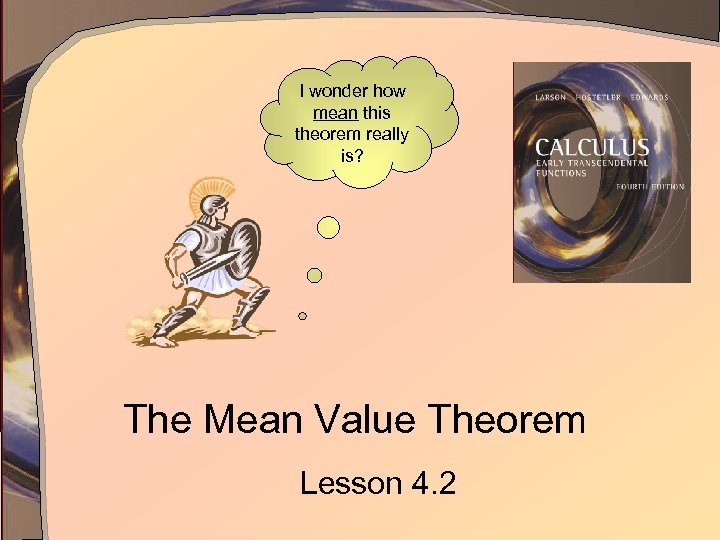I wonder how mean this theorem really is? The Mean Value Theorem Lesson 4. 2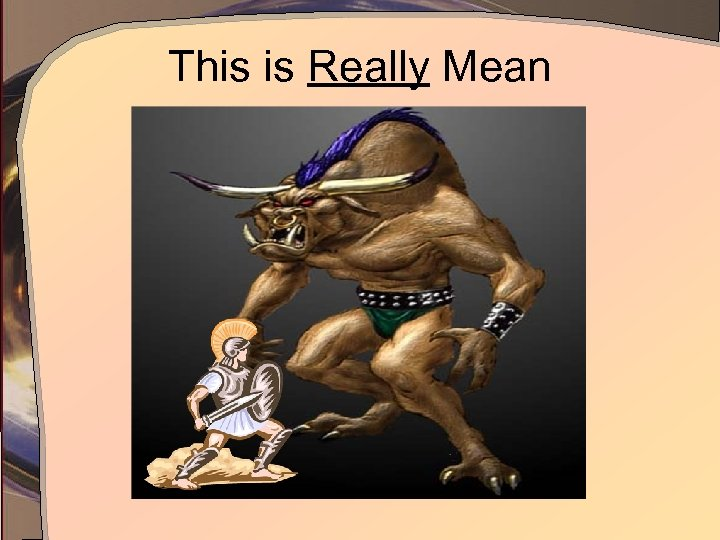This is Really Mean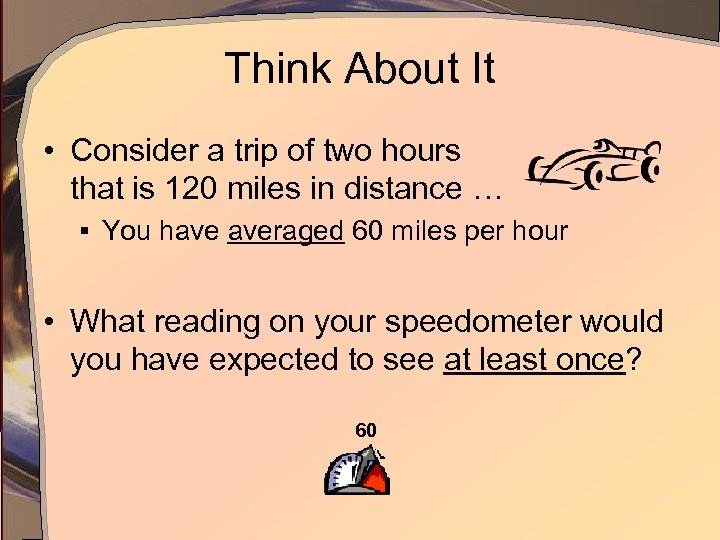Think About It • Consider a trip of two hours that is 120 miles in distance … § You have averaged 60 miles per hour • What reading on your speedometer would you have expected to see at least once? 60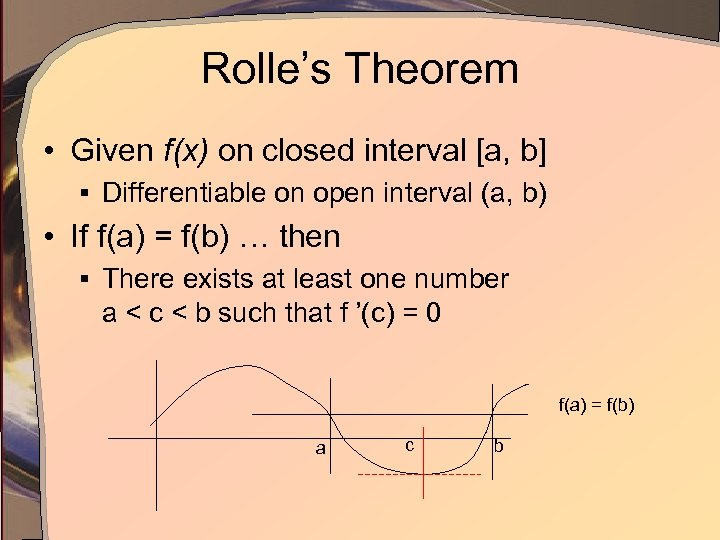Rolle’s Theorem • Given f(x) on closed interval [a, b] § Differentiable on open interval (a, b) • If f(a) = f(b) … then § There exists at least one number a < c < b such that f ’(c) = 0 f(a) = f(b) a c bMean Value Theorem • We can “tilt” the picture of Rolle’s Theorem § Stipulating that f(a) ≠ f(b) • Then there exists a c such that c a b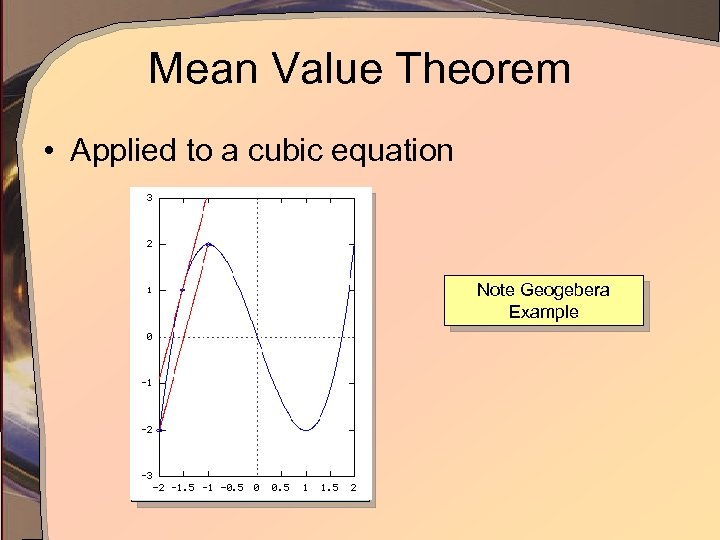Mean Value Theorem • Applied to a cubic equation Note Geogebera Example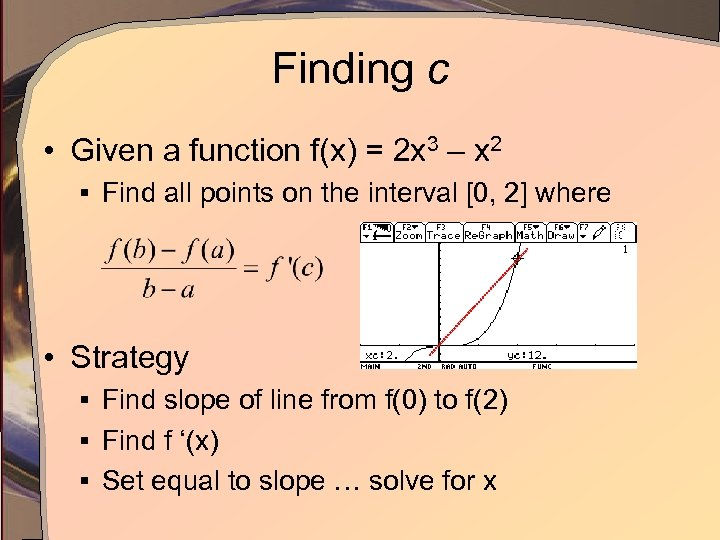Finding c • Given a function f(x) = 2 x 3 – x 2 § Find all points on the interval [0, 2] where • Strategy § Find slope of line from f(0) to f(2) § Find f ‘(x) § Set equal to slope … solve for x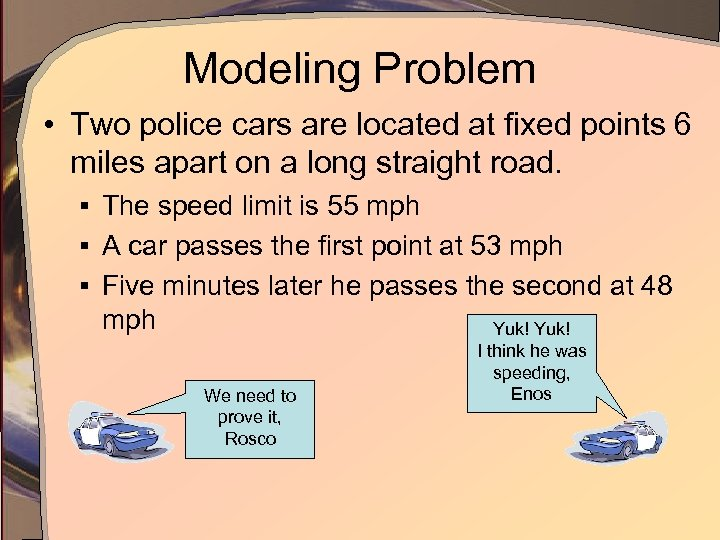Modeling Problem • Two police cars are located at fixed points 6 miles apart on a long straight road. § The speed limit is 55 mph § A car passes the first point at 53 mph § Five minutes later he passes the second at 48 mph We need to prove it, Rosco Yuk! I think he was speeding, Enos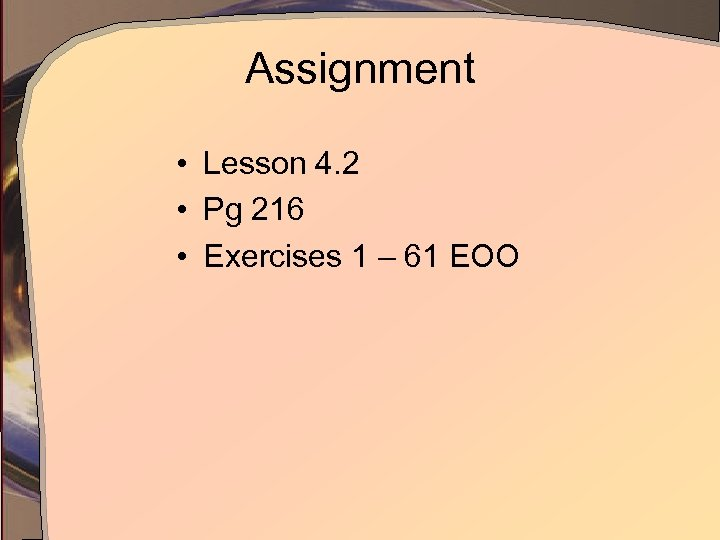Assignment • Lesson 4. 2 • Pg 216 • Exercises 1 – 61 EOO